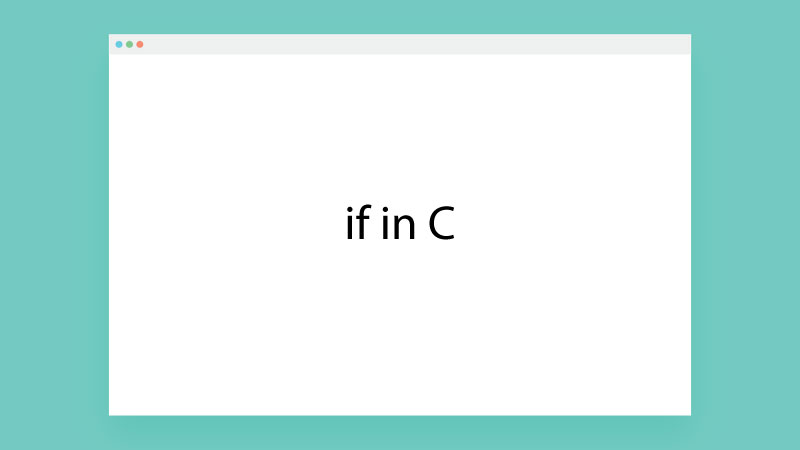# What is if in C Programming

Author: Janet### if in C

In C programming if is a decision-making statement, it checks a single condition whether the condition is true or false. If the condition statement returns true then the compiler executes the scope of if statement and if the condition returns false compiler skips the scope of if statement from being executed.

#### if Statement Syntax

if(condition)

{

// scope of if statement or if block

}

• if statement is used to check only one condition at a time.
• In a single C program, we can use more than one if statement.
• if the condition/expression is true then the scope of the if statement will run.
• if the condition/expression is false then the scope of the if statement will not run.

Example:-

#include<stdio.h>

int main()

{

int m=10, n=2, res;

res=m+n;

if(m>n)

{

printf("m is bigger than n \n");

}

if(n>m)

{

printf("n is bigger than m \n");

}

printf("m+n = %d\n",res);

return 0;

}

Output

Message from if 1

n is big

m+n = 12

#### Combined Condition in if Statement

In if expression more than one condition can be combined together by using logical operators &&, ||, !

Example:-

if(a>b && a<50)

if(a<b || a< 25)

if(!(a>b))

Example:-

A father promises his son that if he is 18+ and gets more than 60% marks in his exam, they will give him a bike, now write a C program that can check whether his son is eligible for the bike or not.

#include<stdio.h>

int main()

{

int age, marks;

printf("Enter age of the student \n");

scanf("%d", &age);

printf("Enter marks of the student \n");

scanf("%d", &marks);

if( (age>18) && (marks>60))

{

printf("Your son is eligible for bike");

}

if(!((age>18) && (marks>60)))

{

printf("Your son is not eligible for bike \n");

}

return 0;

}

Output

Enter age of the student

19

Enter marks of the student

76

Your son is eligible for bike

Note: Logical operator ! is used to converting true condition into false and false into true.

Let's take some real-time problem solutions with if condition in C programming.

#### Program 1

Make a simple arithmetic calculator by using if statement in C programming.

#include<stdio.h>

int main()

{

int n1, n2, n3, res;

printf("Enter the first number \n");

scanf("%d", &n1);

printf("Enter the second number \n");

scanf("%d", &n2);

printf("For + enter 1\n");

printf("For - enter 2\n");

printf("For * enter 3\n");

printf("For / enter 4\n");

scanf("%d", &n3);

if(n3==1)

{

res=n1+n2;

printf("Sum = %d",res);

}

if(n3==2)

{

res=n1-n2;

printf("Sub = %d",res);

}

if(n3==3)

{

res=n1*n2;

printf("Mul = %d",res);

}

if(n3==4)

{

res=n1/n2;

printf("Div = %d",res);

}

return 0;

}

Output

Enter the first number

22

Enter the second number

7

For + enter 1

For - enter 2

For * enter 3

For / enter 4

1

Sum = 29

Note: There are no fixed and hard rules to use if condition in C programs, it depends on you how do you want to use if condition.